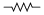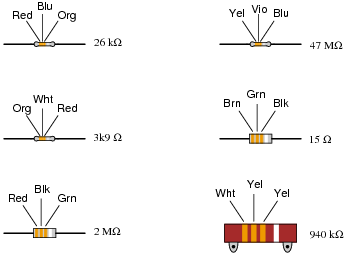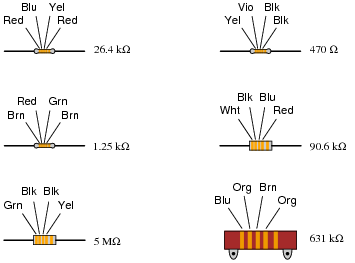# Resistors

## Basic Electricity

• #### Question 1

Shown here is the schematic symbol for a resistor:What is the purpose of a resistor? What function does it perform? Also, draw an illustration of what a real resistor looks like.

• #### Question 2

Resistors are sometimes represented in electrical and electronic schematic diagrams by a symbol other than this:Draw this other symbol next to the one shown above.

• #### Question 3

A primitive resistor may be formed by sketching a thick line on a piece of paper, using a pencil (not an ink pen!):How may the end-to-end electrical resistance of this pencil mark be increased? How may it be decreased? Explain your answers.

• #### Question 4

When a resistor conducts electric current, its temperature increases. Explain how this phenomenon is significant to the application of resistors in electric circuits. In other words, why would we care about a resistor’s temperature increasing?

Also, what does this indicate about the technical ratings of resistors? Aside from having a specific resistance rating (i.e. a certain number of ohms), what other rating is important for proper selection of resistors in electric circuits?

• #### Question 5

Many resistors have their electrical resistance shown by a set of color codes, or “bands,” imprinted around their circumference. A standard color code associates each color with a specific decimal digit (0 through 9). Associate each of the following digits with its respective color:

0 =
1 =
2 =
3 =
4 =
5 =
6 =
7 =
8 =
9 =
• #### Question 6

Observe the following “4-band” resistors, their color codes, and corresponding resistance values (note that the last color band is omitted, since it deals with precision and not nominal value):What patterns do you notice between the color codes (given as three-letter abbreviations, so as to avoid interpretational errors resulting from variations in print quality), the resistance values, and the physical sizes of the resistors?

• #### Question 7

What does the last color band represent on a color-coded resistor?

• #### Question 8

Determine the nominal resistance values of these resistors, given their band colors, and also express the allowable tolerance in ohms.

For example, a 25 kΩ resistor with a 10% tolerance rating would have an allowable tolerance of /- 2.5 kΩ.

• Red, Org, Blu, Gld =
• Brn, Blk, Grn, Sil =
• Blu, Blk, Brn, Gld =
• Yel, Vio, Red, Sil =
• Grn, Brn, Yel =
• Wht, Blu, Blk, Sil =
• Gry, Grn, Org, Gld =
• Org, Org, Gld =
• Vio, Red, Sil, Gld =
• Brn, Red, Blk, Sil =
• #### Question 9

Observe the following “5-band” precision resistors, their color codes, and corresponding resistance values (note that the last color band is omitted, since it deals with precision and not nominal value):What patterns do you notice between the color codes (given as three-letter abbreviations, so as to avoid interpretational errors resulting from variations in print quality) and the resistance values of each resistor? Why do precision resistors use a “5-band” color code instead of a “4-band” color code?

• #### Question 10

Determine whether or not the following resistors measure within the resistance range specified by their color codes:

• (Org, Org, Red, Blk, Blu) Measured resistance = 332.5 Ω
• (Brn, Blk, Blk, Gld, Red) Measured resistance = 9.7 Ω
• (Blu, Vio, Brn, Red, Grn) Measured resistance = 67.43 kΩ
• (Red, Wht, Grn, Yel, Vio) Measured resistance = 2.949 MΩ
• (Yel, Vio, Org, Gld) Measured resistance = 44.68 kΩ
• (Gry, Red, Brn, Sil) Measured resistance = 905 Ω
• (Grn, Blu, Gld) Measured resistance = 6.73 Ω
• (Vio, Brn, Red, Gld, Brn) Measured resistance = 70.82 Ω
• (Wht, Org, Blu, Brn, Grn) Measured resistance = 9.38 kΩ
• (Red, Blk, Wht, Grn, Vio) Measured resistance = 20.86 MΩ

Assume that all five-band resistors listed here use the precision color code as opposed to the military 5-band code where the fifth band indicates resistor reliability.

• #### Question 11

Find one or two real resistors and bring them with you to class for discussion. Identify as much information as you can about your resistors prior to discussion:

• Resistance (ideal)
• Resistance (actual)
• Power rating
• Type (carbon composition, metal film, wire-wound, etc.)

### Related Content

Published under the terms and conditions of the Creative Commons Attribution License•Kuronikuruph July 13, 2020
•Kuronikuruph July 13, 2020